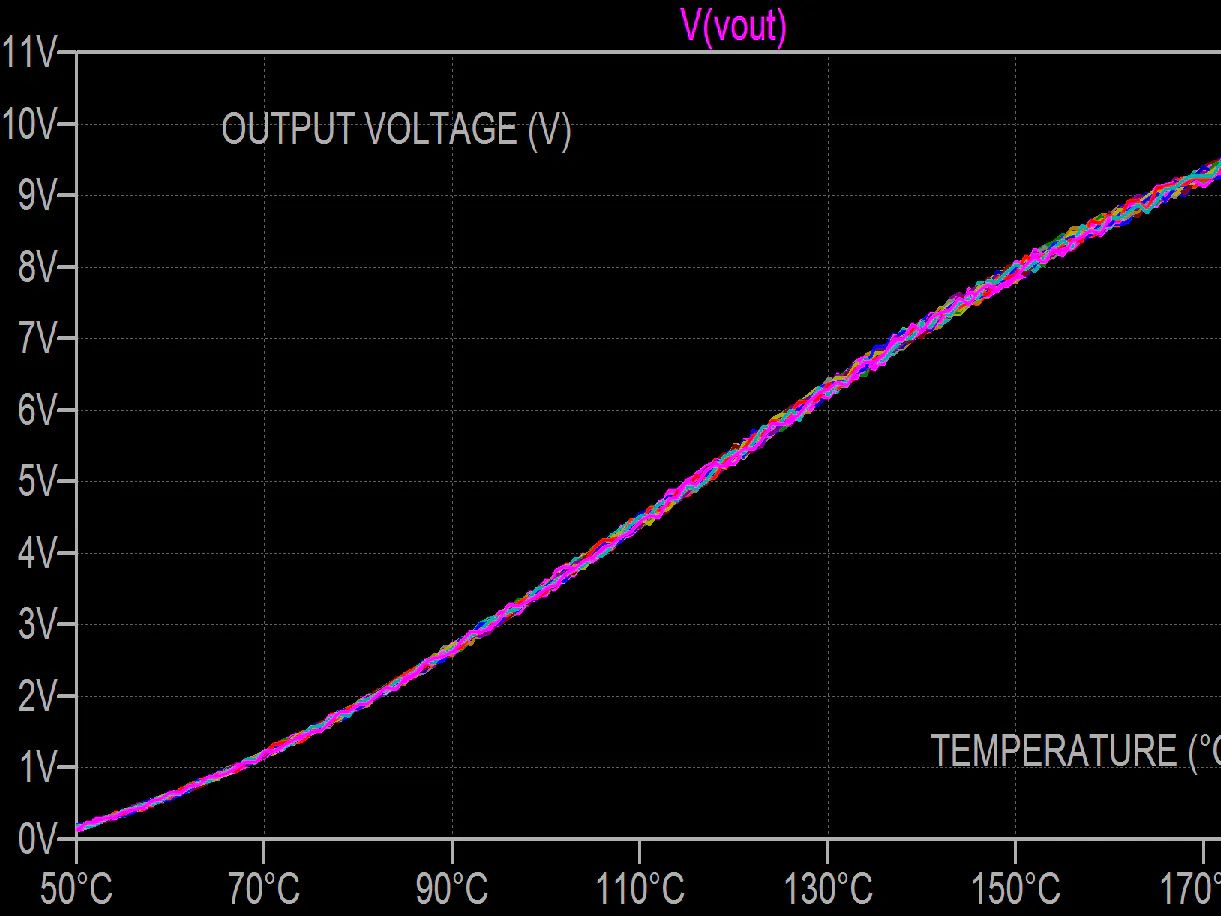# Oil temperature measurement simulation with Vishay NTCLE350

A oil temperature measurement circuit is simulated in LTspice of a wheatstone bridge with a thermistor for temp. between 50 and 185°C.

BeginnerProtip1 hour10## Things used in this project

### Hardware components

 Vishay NTCLE350E4303FHB0
×1
 Vishay CRCW
×1
×1

## Schematics

### LTspice simulation NTCLE350 oil temperature sensing

extract archive and open it in LTspice XVII

## Code

### netlist for a Vishay NTC themistor NTCLE350 with temperature driven by voltage

Plain text
it's a netlist usable in LTspice XVII
```* Voltage_driven_thermistor NTCLE350
* Vishay Intertechnology, Inc.
* customer : general
* Revision:   1.0  \$
* Author:   Alain Stas  \$
* Date:   13 June 2020 \$
* Revision History :correction <> introduced
*---------------------------------------------------------------
* data sheet :https://www.vishay.com/docs/29218/ntcle350e4.pdf
* the different R25 and B are as follows : NTCLE350E4103_HB0   = 10Kohms B25/85=3984K
*                                                    103_LB0 = 10Kohms       =3435K
*                                                    212_MB0  = 12Kohms       =3514 K
*                                                    303_HB0  = 30 Kohms       =3935K
*                                                    502_LB0   = 5Kohms       =3435K
* tolerance on R25 and Steinhart & Hart coefficients modelled with Monte Carlo distribution
*
*                electrical pins (to be connected to electrical circuit)
*\$                |  |
*                 |  | temperature pin ( for simulation only ,to be connected to temperature-voltage)
*                 |  | |
.SUBCKT NTC_BASE RN Rp Ti Params: W=1 X=1 Y=1 Z=1 GTH=1 GTH1=1 CTH=1 A=1 R25=1 B=1 C=1 D=1 T0=273.15 TRmc=5 TBmc=1
R_RTR    0  TR  {mc(1,TR-1)}
I_ITR    0 TR 1
R_RTB    0 TB  {mc(1,TB-1)}
I_ITB    0 TB  1
G_G1         AOUT 0 VALUE { if(V(Ti)>25,V(AOUT,
+  0)/(R25*V(TR)*exp(((D*V(TB)/(T0+abs(V(H))+V(Ti))+C*V(TB))/(T0+abs(V(H))+V(Ti))+B*V(TB))/(T0+V(Ti)+abs(V(H)))+A*V(TB))),0)
+  }
G_G2         AOUT 0 VALUE { IF(V(Ti)>25,0,V(AOUT,
+  0)/(R25*V(TR)*exp(((Z*V(TB)/(T0+abs(V(H))+V(Ti))+Y*V(TB))/(T0+abs(V(H))+V(Ti))+X*V(TB))/(T0+abs(V(H))+V(Ti))+W*V(TB))))
+  }
G_G3         H 0 VALUE {
+  if(V(Ti)>25,-V(RP,RN)*V(RP,RN)/(R25*V(TR)*exp(((D*V(TB)/(T0+abs(V(H))+V(Ti))+C*V(TB))/(T0+abs(V(H))+V(Ti))+B*V(TB))/
+  (T0+V(Ti)+abs(V(H)))+A*V(TB))),0)}
G_G4         H 0 VALUE {
+  if(V(Ti)>25,0,-V(RP,RN)*V(RP,RN)/(R25*V(TR)*exp(((Z*TB/(T0+abs(V(H))+V(Ti))+Y*V(TB))/(T0+abs(V(H))+V(Ti))+X*V(TB))/
+  (T0+V(Ti)+abs(V(H)))+W*V(TB))))}
G_G5         RP RN VALUE { V(RP, RN)/V(AOUT) }
G_G6         H 0 VALUE { V(H)*(Gth + Gth1*(V(Ti)-25)) }
R_R1         0 AOUT  1T TC=0,0
R_R2         0 H  1T TC=0,0
C_C1         0 H  {Cth}
R_R99        0 Ti 1T
.IC V(H) =0
.ENDS
.SUBCKT NTC2_BASE RN Rp Ti Params: W=1 X=1 Y=1 Z=1 GTH=1 GTH1=1 CTH=1 A=1 R25=1 B=1 C=1 D=1 T0=273.15 TRmc=5 TBmc=1
R_RTR    0  TR  {mc(1,TR-1)}
I_ITR    0 TR 1
R_RTB    0 TB  {mc(1,TB-1)}
I_ITB    0 TB  1
G_G7         AOUT 0 VALUE { if((V(Ti)>=150),V(AOUT,
+  0)/(53.68316*V(TR)*exp(3843*(1/(V(Ti)+abs(V(H))+T0)-1/423.15))),0)*(1 +(V(TB)-1)*3528*(1/(T0+abs(V(H))+V(Ti))-1/298.15))
+  }
G_G1         AOUT 0 VALUE { if((V(Ti)>25)&(V(Ti)<150),V(AOUT,
+  0)/(R25*V(TR)*exp(((D*V(TB)/(T0+abs(V(H))+V(Ti))+C*V(TB))/(T0+abs(V(H))+V(Ti))+B*V(TB))/(T0+V(Ti)+abs(V(H)))+A*V(TB))),0)
+  }
G_G2         AOUT 0 VALUE { IF(V(Ti)>25,0,V(AOUT,
+  0)/(R25*V(TR)*exp(((Z*V(TB)/(T0+abs(V(H))+V(Ti))+Y*V(TB))/(T0+abs(V(H))+V(Ti))+X*V(TB))/(T0+abs(V(H))+V(Ti))+W*V(TB))))
+  }
G_G3         H 0 VALUE {
+  if(V(Ti)>25,-V(RP,RN)*V(RP,RN)/(R25*V(TR)*exp(((D*V(TB)/(T0+abs(V(H))+V(Ti))+C*V(TB))/(T0+abs(V(H))+V(Ti))+B*V(TB))/
+  (T0+V(Ti)+abs(V(H)))+A*V(TB))),0)}
G_G4         H 0 VALUE {
+  if(V(Ti)>25,0,-V(RP,RN)*V(RP,RN)/(R25*V(TR)*exp(((Z*TB/(T0+abs(V(H))+V(Ti))+Y*V(TB))/(T0+abs(V(H))+V(Ti))+X*V(TB))/
+  (T0+V(Ti)+abs(V(H)))+W*V(TB))))}
G_G5         RP RN VALUE { V(RP, RN)/V(AOUT) }
G_G6         H 0 VALUE { V(H)*(Gth + Gth1*(V(Ti)-25)) }
R_R1         0 AOUT  1T TC=0,0
R_R2         0 H  1T TC=0,0
C_C1         0 H  {Cth}
R_R99        0 Ti 1T
.IC V(H) =0
.ENDS
*\$
.SUBCKT NTCLE350E4103_LB0 RN Rp Ti  PARAMS: TOLR=0 TOLB=0
X45 RN Rp Ti NTC_BASE Params:
+ w=-12.8922832
+ x=4245.148
+ y=-87493
+ z=-9588114
+ gth=0.0015 gth1 = 0.00000
+ cth=0.018
+ a=-12.89228328
+ r25=10000
+ b=4245.148
+ c=-87493
+ d=-9588114
+ T0=273.15
+ TR={1+TOLR/100}
+ TB={1+TOLB/100}
.ENDS
*\$
.SUBCKT NTCLE350E4103_HB0 RN Rp Ti  PARAMS: TOLR=0 TOLB=0
X46 RN Rp Ti NTC_BASE Params:
+ w=-14.6571976
+ x=4798.842
+ y=-115334
+ z=-3730535
+ gth=0.0015 gth1 = 0.00000
+ cth=0.018
+ a=-14.65719769
+ r25=10000
+ b=4798.842
+ c=-115334
+ d=-3730535
+ T0=273.15
+ TR={1+TOLR/100}
+ TB={1+TOLB/100}
.ENDS
*\$
.SUBCKT NTCLE350E4502_LB0 RN Rp Ti  PARAMS: TOLR=0 TOLB=0
X RN Rp Ti NTC_BASE Params:
+ w=-12.8922832
+ x=4245.148
+ y=-87493
+ z=-9588114
+ gth=0.0015 gth1 = 0.00000
+ cth=0.018
+ a=-12.89228328
+ r25=5000
+ b=4245.148
+ c=-87493
+ d=-9588114
+ T0=273.15
+ TR={1+TOLR/100}
+ TB={1+TOLB/100}
.ENDS
*\$
*\$
.SUBCKT NTCLE350E4212_MB0 RN Rp Ti  PARAMS: TOLR=0 TOLB=0
X42 RN Rp Ti NTC2_BASE Params:
+ w=-12.002581720610
+ x=3670.667
+ y=-7617.13
+ z=-5914730
+ gth=0.0015 gth1 = 0.00000
+ cth=0.018
+ a=-21.0133817206104
+ r25=2100
+ b=11886.95
+ c=-2504699
+ d=247033800
+ T0=273.15
+ TR={1+TOLR/100}
+ TB={1+TOLB/100}
.ENDS
*\$
.SUBCKT NTCLE350E4303_HB0 RN Rp Ti  PARAMS: TOLR=0 TOLB=0
X53 RN Rp Ti NTC_BASE Params:
+ w=-14.5513561
+ x=4752.725
+ y=-98275
+ z=-7522357
+ gth=0.0015 gth1 = 0.0000
+ cth=0.010
+ a=-14.55135614
+ r25=30000
+ b=4752.725
+ c=-98275
+ d=-7522357
+ T0=273.15
+ TR={1+TOLR/100}
+ TB={1+TOLB/100}
.ENDS
```

## Credits

### alainstas

13 projects • 2 followers
product engineer at Vishay